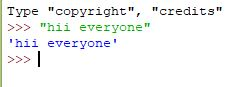# Input and Output

• Difficulty Level : Basic
• Last Updated : 07 Jun, 2022

Let us discuss what is Console in Python. Console (also called Shell) is basically a command-line interpreter that takes input from the user i.e one command at a time and interprets it. If it is error-free then it runs the command and gives the required output otherwise shows the error message. A Python Console looks like this.Here we write a command and to execute the command just press enter key and your command will be interpreted. For coding in Python, you must know the basics of the console used in Python. The primary prompt of the python console is the three greater than symbols:

`>>>`

You are free to write the next command on the shell only when after executing the first command these prompts have appeared. The Python Console accepts commands in Python that you write after the prompt.Accepting Input from Console User enters the values in the Console and that value is then used in the program as it was required. To take input from the user we make use of a built-in function input()

Example

## Python

 `# input``input1 ``=` `input``()` `# output``print``(input1)`

Output:

```>>>Geeks for Geeks
'Geeks for Geeks'```

We can also type cast this input to integer, float or string by specifying the input() function inside the type.

Typecasting the input to Integer: There might be conditions when you might require integer input from user/Console, the following code takes two input(integer/float) from console and typecasts them to integer then prints the sum.

## Python

 `# input``num1 ``=` `int``(``input``())``num2 ``=` `int``(``input``())` `# printing the sum in integer``print``(num1 ``+` `num2)`

Output:

```>>>10
>>>20
30```

Typecasting the input to Float: To convert the input to float the following code will work out.

## Python

 `# input``num1 ``=` `float``(``input``())``num2 ``=` `float``(``input``())` `# printing the sum in float``print``(num1 ``+` `num2)`

Output:

```>>>10
>>>20
30.0```

Typecasting the input to String: All kinds of input can be converted to string type whether they are float or integer. We make use of keyword str for typecasting.

## Python

 `# input``string ``=` `str``(``input``())` `# output``print``(string)`

Output:

```>>>20.0
'20.0'```

How to Input Multiple Values From User in One Line: For instance, in C we can do something like this:

## C

 `// Reads two values in one line``scanf``("%d %d", &x, &y)`

One solution is to use input() function two times.

## Python3

 `x, y ``=` `input``(), ``input``()``print``(``"x="``,x,``"y="``,y)`

Output:

```>>>geeks
>>>for geeks
x= geeks y= forgeeks ```

Another solution is to use split() function.

## Python3

 `x, y ``=` `input``().split()``print``(``"x="``,x,``"y="``,y)`

Output:

```>>>10 20
x= 10 y= 20```

Note that we don’t have to explicitly specify split(‘ ‘) because split() uses whitespace characters as a delimiter as default. One thing to note in the above Python code is, that both x and y would be of string. We can convert them to int using another line.

```>>>x, y = [int(x), int(y)]
>>>print(x,y)
10 20

# We can also use  list comprehension
>>>x, y = [int(x) for x in [x, y]]
>>>print(x,y)
10 20```

Below is a complete one-line code to read two integer variables from standard input using split and list comprehension.

## Python3

 `# Read two numbers from input and typecasts them to int using``# list comprehension``x, y ``=` `[``int``(x) ``for` `x ``in` `input``().split()] `

## Python3

 `# Reads two numbers from input and typecasts them to int using``# map function``x, y ``=` `map``(``int``, ``input``().split())`

END Parameter: By default python’s print() function ends with a newline. A programmer with C/C++ background may wonder how to print without a newline. Python’s print() function comes with a parameter called ‘end’. By default, the value of this parameter is ‘\n’, i.e., the new line character. You can end a print statement with any character/string using this parameter.

## Python3

 `# This Python program must be run with``# Python 3 as it won't work with 2.7.` `# ends the output with a ``print``("Welcome to" , end ``=` `' '``)``print``("GeeksforGeeks", end ``=` `' '``)`

Output:

`Welcome to GeeksforGeeks`

One more program to demonstrate the working of end parameter.

## Python3

 `# This Python program must be run with``# Python 3 as it won't work with 2.7.` `# ends the output with '@'``print``("Python" , end ``=` `'@'``)``print``("GeeksforGeeks")`

Output:

`Python@GeeksforGeeks`

My Personal Notes arrow_drop_up